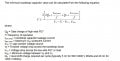# Bootstrap Capacitor Calculation for MOSFET Gate Driver help

#### jlawley1969

Joined Feb 22, 2021
97
Hello,
I am trying to calculate the minimum capacitor size in a bootstrap for an IRS21867 gate driver. I have included the equation by IR's application notes. What I am not getting are the values of Vls and Vmin.If I am inverting 100V that means Vls would 100V? because that would be the drop across the lowside FET as its S is connected to ground. This its self is not the issue(unless I am wrong in my assumption) the issue is if I for example have Vcc = 15V and Vf = 0.7V that would give me a negative value in the denominator and thus a negative capacitance overall.

As a second smaller question while I am here, what is Vmin in this case? does it have to do with under voltage specification between these two pins on the IC or is it simply 0V?

I have built the circuit in real life and tested capacitors I had on hand and they all worked fine, I just want to know if I can buy smaller / I got very frustrated trying to solve this equation as it is poorly defined and I was getting illogical answers. Thanks as always.

Here is the application note link:
http://www.farnell.com/datasheets/2254418.pdf

#### mohit.2

Joined May 16, 2021
1
Hello,
I am trying to calculate the minimum capacitor size in a bootstrap for an IRS21867 gate driver. I have included the equation by IR's application notes. What I am not getting are the values of Vls and Vmin. View attachment 241466
If I am inverting 100V that means Vls would 100V? because that would be the drop across the lowside FET as its S is connected to ground. This its self is not the issue(unless I am wrong in my assumption) the issue is if I for example have Vcc = 15V and Vf = 0.7V that would give me a negative value in the denominator and thus a negative capacitance overall.

As a second smaller question while I am here, what is Vmin in this case? does it have to do with under voltage specification between these two pins on the IC or is it simply 0V?

I have built the circuit in real life and tested capacitors I had on hand and they all worked fine, I just want to know if I can buy smaller / I got very frustrated trying to solve this equation as it is poorly defined and I was getting illogical answers. Thanks as always.

Here is the application note link:
http://www.farnell.com/datasheets/2254418.pdf
Vmin
It is the high side under voltage lockout .
Refer the Gate drive datasheet .